MATERIALS TRANSACTIONS
Online ISSN : 1347-5320
Print ISSN : 1345-9678
ISSN-L : 1345-9678
Microstructure of Materials
High Temperature Deformation and Microstructure Evolution of Ni–Co Base Superalloy TMW-4M3
Takaaki HaraToshiki IshidaKatsunari Oikawa

ジャーナル フリー HTML

2020 年 61 巻 4 号 p. 632-640Browse “Advance online publication” version

Abstract

The high temperature deformation and microstructure evolution of Ni–Co base superalloy TMW-4M3 during the isothermal forging process were studied. A uniform compression test of TMW-4M3 where both the strain rate and compression temperature were controlled showed dynamic recrystallization flow stress. The peak stress and steady stress of the deformation resistance curve were characterized with the Zener-Hollomon parameter. The average grain size after dynamic recrystallization was also correlated with the Zener-Hollomon parameter, but this relationship changed with compression temperature. We found that this temperature dependency was related to the pinning effect of the γ′ precipitates in the γ matrix and proposed a new prediction model for dynamic recrystallization grain size considering not only the Zener-Hollomon parameter but also the volume fraction of the γ′ precipitates. This enables us to calculate the average grain size after isothermal forging within an error of 12%.Fig. 14 Prediction accuracy of developed numerical model for dynamic recrystallization grain size considering pinning effect.

1. Introduction

The turbine disks used in the jet engines or power generators are mainly made of cast and wrought Ni-base superalloys because of their high heat resistance and high strength properties. The temperature in a combustion chamber determines the performance of the gas turbines. Therefore, a lot of novel superalloys are constantly being developed to improve the service temperature. The high temperature strength property of the Ni-base superalloy is caused by the γ′ precipitates, which prevent dislocation migration during deformation. In the case of turbine blades, single crystal Ni-base superalloys are used, and the volume fraction of the γ′ phase is about 70%. On the other hand, the microstructure of the turbine disks is usually designed as fine grain structure to obtain a good low cycle fatigue property. This fine grain structure was achieved by dynamic recrystallization during the hot processing. If the alloy has a much larger amount of γ′ precipitates, the workability decreases although the high temperature strength property is improved. The turbine disks usually have complex shapes and are manufactured by forging process. Therefore, we need to take into account both the required material properties and good manufacturability.

TMW-4M3 is a superalloy that was developed by the National Institute for Material Science in Japan.1,2) This Ni–Co base superalloy was designed to have good phase stability and thermal resistance by adding Co and Ti to Alloy 720Li. The nominal compositions of TMW-4M3 and Alloy 720Li are shown in Table 1. Since the Ti composition is higher than that of Alloy 720Li, the amount of γ′ phase increases, which has a precipitation strengthening effect. The maximum volume fraction of the γ′ phase is about 50% for TMW-4M3 and 45% for Alloy 720Li.Table 1 Nominal compositions of TMW-4M3 and Alloy 720Li (mass%).

To apply TMW-4M3 to turbine disks, we have to design the forging process. Generally, the hot forging process is applied to these high strength superalloys which have a large amount of γ′ precipitates. In this process, the material is preliminarily heated to decrease its deformation resistance. However, during the forging process, the material temperature decreases due to the material being in contact with the forging dies. The temperature drop during the forging process causes γ′ precipitation, which leads to an increase of deformation resistance. One solution to this is isothermal forging, where the forging dies are kept at the same temperature as the forging material, and we can forge the target material with relatively low deformation resistance compared to the conventional hot forging process. On the other hand, both an active grain boundary migration and a smaller volume fraction of γ′ precipitates are led by too high temperature processing. This situation often causes grain coarsening, which results in lower tensile strength and low cycle fatigue properties.

Prior to the trial and error approach of prototype production, numerical modeling and simulation was used as a very strong tool to find the optimum forging conditions because the prototype production of large forged parts such as turbine disks cost too much. For example, dynamic recrystallization behavior during the hot deformation process and the resulting microstructure has usually been studied by the relationship with the Zener-Hollomon parameter in many Ni-base superalloys.35) On the other hand, the effect of γ′ precipitates on recrystallization are only considered qualitatively.5,6)

The objective of this work is to investigate the high temperature deformation property and the dynamic recrystallization behavior of TMW-4M3 using isothermal compression tests and to reveal the relationship between the forging parameters and the microstructures under the effect of γ′ precipitates.

2. Experimental Methods

2.1 Material and sample preparation

The chemical composition of TMW-4M3 used in this study is listed in Table 2. The as-received alloy was a wrought billet, and cylindrical specimens were cut from the middle part of the billet, along the longitudinal direction. They are 12 mm in height and 8 mm in diameter. Microstructures observed by optical microscope are shown in Fig. 1. The shapes of the γ′ particles were clearly indicated by etching in Kalling’s solution. We found that the effect of solute segregation in the casting process was sufficiently small because of the uniform distribution of the γ′ precipitates. The average grain size of the matrix γ phase was about 18–20 µm.Table 2 Chemical composition of TMW-4M3 specimens (mass%).Fig. 1

Optical micrographs of TMW-4M3 specimen with (a) low magnification and (b) high magnification. The γ′ particles are uniformly distributed.

2.2 Uniform and isothermal compression test

Compression tests were conducted to reproduce isothermal forging conditions using a THERMECMASTOR-Z thermo-mechanical simulator.7,8) We inserted dummy metallic dies between the specimen and the SiC anvils and heated the specimen with a surrounding induction heating coil to control the material temperature. Although the ceramic anvils were not heated, the dummy dies were heated together with the specimen. Therefore, the heat transfer from the specimen to the anvils was extremely reduced, and the isothermal compression test could be performed. In this experiment, we used Nimowal alloy as the dummy dies’ material.9,10) In addition, we inserted glass sheets between the dummy dies and the specimen and moreover made concentric grooves on the upper and lower surfaces of the cylindrical specimens. When the dummy dies and specimens are heated, melted glass enters the grooves and acts as a lubricant during compression. Then, we can evaluate the exact loading data of the material due to the reduced friction at the upper and lower surfaces of the compression specimen.

As for the compression conditions, the deformation temperature ranged from 1223 to 1433 K, and the strain rate ranged from 0.0005 to 0.05 s−1. All specimens were heated to the deformation temperature and soaked for 10 min to achieve uniform temperature distributions and equlibrium state of the temperature. Just after the specimens were compressed at the target temperature and strain rate, they were quenched by N2 gas to keep the deformed microstructure. During the whole of the compression process, we monitored the temperature history of the specimens with thermocouples connected to their surfaces and controlled the power of the induction heating coil so as to maintain the isothermal conditions.

The flow stress curve of TMW-4M3 was calculated from the load curve measured during the compression test. Moreover, we cut the compressed specimens parallel to the compression axis through the center. The surfaces of the cut samples were polished with colloidal silica and etched in Kalling’s solution. Micrographs of the samples were taken at around the center of the etched surface using an optical microscope. These observation points were decided by finite element analysis, which enables us to select points whose temperature and strain rate history were relatively stable through compression. The matrix grain size was evaluated by planimetric method. The volume fraction of γ′ precipitates of the SEM image was quantitatively analyzed using Image-Pro Plus software.11)

3. Results and Discussions

3.1 Deformation behavior

We calculated the true stress-strain curves of TMW-4M3 from actual load-stroke data obtained from compression tests. The flow stress curves in Fig. 2 show dynamic recrystallization behavior, which has peak stress σp and steady stress σs. Peak stress σp is the maximum point of flow stress and originates from the balance between work hardening by accumulation of dislocation and softening by dynamic recovery and recrystallization. After the peak stress point, dynamic recrystallization becomes predominant relative to work hardening, and flow stress decreases. Steady stress σs is the stable value of the flow stress curve in the larger strain range. In this region, the whole of the matrix phase is composed of recrystallized grains, and introduced dislocation by deformation is constantly balanced with dislocation annihilation by dynamic recrystallization. The relationship between these characteristic stresses and compression conditions in the precipitation strengthening type Ni-base superalloys is given as the following numerical modeling,

 \begin{equation} Z = \dot{\varepsilon}\exp (Q/RT) = A\sigma^{n'}, \end{equation} (1)
where Z is the Zener-Hollomon parameter that denotes the temperature-compensated strain rate, $\dot{\varepsilon }$ is the strain rate, Q is the activation energy of high temperature deformation, R is the gas constant, T is the deformation temperature, σ is the flow stress, and A and n′ are the material constants.12,13) Then, we evaluated the process dependency of σp and σs using eq. (1).Fig. 2

Flow stress curve of TMW-4M3 at 1223 K.

First, the temperature dependency of σp and σs were investigated. We took the natural log of eq. (1) and obtained the following expression,

 \begin{equation} \ln \dot{\varepsilon} + (Q/R)(1/T) = \ln A + n'\ln \sigma. \end{equation} (2)
Under constant strain rate conditions, we can evaluate the effect of activation energy from the slope of the Arrhenius plot for σ using eq. (2). Figure 3 shows the Arrhenius plots toward σp and σs. For the strain rates of 0.05 s−1 and 0.005 s−1, we found linear relationships. In the case of the 0.0005 s−1 strain rate, the temperature dependency is different. At high temperature, σp and σs were almost constant, but they increased at low temperature. The slopes Q/nR of all strain rate conditions estimated by the method of least squares are also listed in Fig. 3. In the lower temperature range below 1373 K, the slopes for the strain rate of 0.0005 s−1 had the same order of magnitude as the slope of 0.05 and 0.005 s−1. However, in the higher temperature range greater than or equal to 1373 K, the slope for the strain rate of 0.0005 s−1 was smaller than the other slopes by an order of magnitude. The reduction of the slope implies a decrease of activation energy. The small activation energy is revealed especially in conditions of smaller strain rates and higher compression temperature. Therefore, the dynamic recovery mechanism was assumed to be dominant in this region rather than dynamic recrystallization.Fig. 3

Temperature dependency of flow stress with (a) peak stress and (b) steady stress.

Secondly, stress index n′ was investigated from the relationship between $\ln \dot{\varepsilon }$ and ln σ for each compression temperature shown in Fig. 4. As with Fig. 3, the slope of the approximate straight line in this relationship denotes stress index n′. The calculated values of index n′ for all compression conditions are also written in Fig. 4. Stress index for pure metal and alloys usually ranged from 3 to 7 in previous research, and that for TMW-4M3 was generally consistent with this tendency.7)Fig. 4

Relationship between strain rate and flow stress with (a) peak stress and (b) steady stress.

From the slopes shown in Fig. 3 and Fig. 4, we found that the activation energies in different compression conditions differ. Calculation results of activation energies based on the peak stress and stready stress for all conditions are summarized in Table 3 and Table 4, respectively. These variations in activation energies are thought to be due to the γ′ state change with time and temperature. For example, at a lower temperature, the higher volume fraction of γ′ precipitates increase deformation resistance, which will lead to a large activation energy of deformation. In fact, it has been reported that the activation energy of high temperature deformation in Alloy 720Li also differs according to compression conditions.14) As shown here, in the case of dual phase structure, the microstructure evolution of the matrix phase is affected by the other phase, and the influence rate differs according to the process conditions. In this study, the best fit value of activation energy for the ranges of compression conditions was derived from the average value of the slope in both Fig. 3 and Fig. 4. As for the strain rate of 0.0005 s−1 in Fig. 3, the slopes Q/nR defined from whole temperature range were used: 20050 K·mol for the peak stress, and 13275 K·mol for the steady stress. Then, we obtained 18843 K·mol and 13552 K·mol as the the average values of Q/nR for the peak stress and the steady stress, respectively. Moreover, the average values of n′ derived from Fig. 4 were 3.92 and 3.64 for the peak stress and the steady stress respectively. Finally, we found the activation energies Q as 613.6 kJ for the peak stress and 410.3 kJ for the steady stress. As a result, we developed the following prediction model of σp and σs using the Zenner-Hollomon parameter on the basis of eq. (1).

 \begin{equation} [\sigma_{\text{p}}]\quad Z = \dot{\varepsilon}\exp \left(\frac{613.6 \times 10^{3}}{RT} \right) = 1.07 \times 10^{14} \times \sigma_{\text{p}}^{3.92}, \end{equation} (3)

 \begin{equation} [\sigma_{\text{s}}]\quad Z = \dot{\varepsilon}\exp \left(\frac{410.3 \times 10^{3}}{RT} \right) = 2.19 \times 10^{7} \times \sigma_{\text{s}}^{3.64}. \end{equation} (4)
Here, the material constant A was derived from ln Z-intercept of the approximate straight line in the relationship between ln Z and ln σ. The comparison between the experiments and prediction model are shown in Fig. 5. For each flow stress, the prediction model shows good agreement. Generally, the activation energy of high temperature deformation with dynamic recrystallization is larger than that of diffusion for solute elements. For example, the activation energy of nickel self-diffusion is 281 kJ/mol, and the activation energy of molybdenum inter-diffusion in nickel is 288 kJ/mol. On the other hand, the reported activation energy of high temperature deformation for the Ni-base superalloy Waspaloy ranged from 346 to 468 kJ/mol.5,1517) As for the solute elements in TMW-4M3, the activation energies of aluminum and titanium volume diffusion in nickel are about 260 kJ/mol.1820) Moreover, the activation energies of volume diffusion for cobalt, chromium and tungsten range from 280 to 310 kJ/mol.18,20) Therefore, as with Waspaloy, the activation energies of high temperature deformation for TMW-4M3 are larger than those of volume diffusion for its solute elements, which indicates the deformation by dynamic recrystallization.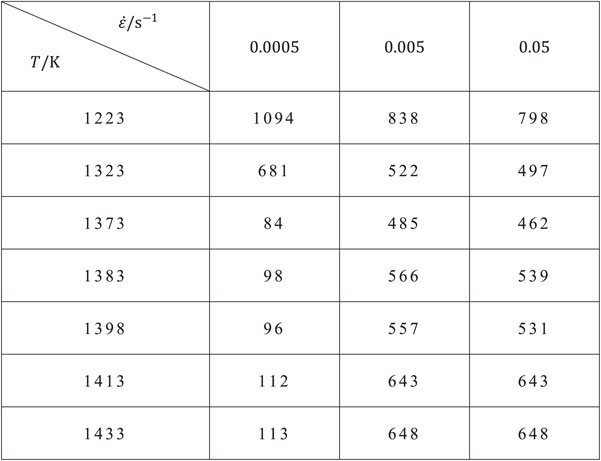Table 3 Calculated activation energy Q of all compression conditions based on the peak stress (kJ).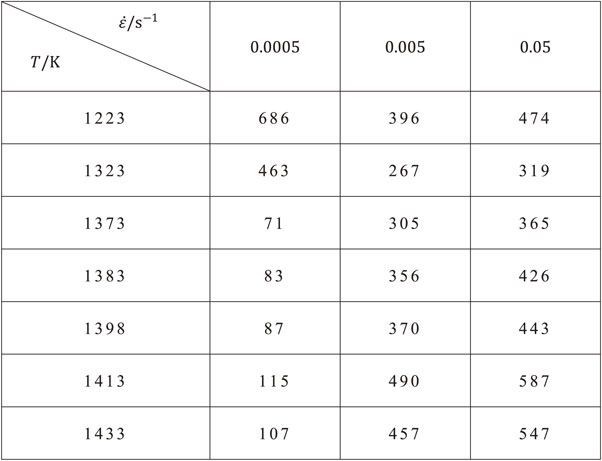Table 4 Calculated activation energy Q of all compression conditions based on the steady stress (kJ).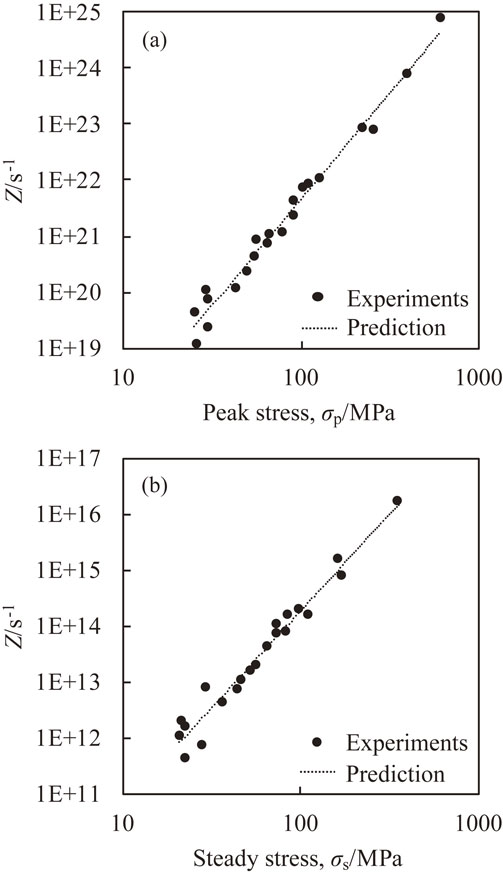Fig. 5

Relationship between Zener-Hollomon parameter and flow stress of TMW-4M3 with (a) peak stress and (b) steady stress.

3.2 Microstructures and pinning effect of γ′ precipitates

Typical microstructures after isothermal compression tests are shown in Fig. 6. The true strains of all test pieces were about 1.4, which showed steady flow stress, and then all the matrix grains were dynamically recrystallized ones. The average grain size after dynamic recrystallization DDRX increased with higher compression temperature. At the same time, the amount of γ′ particles decreased. Moreover, the strain rate dependency of DDRX is indicated in Fig. 7. A faster strain rate resulted in a fine microstructure, and a slower strain rate resulted in a coarse one. Then, the compression temperature dependency of DDRX evaluated by an Arrhenius plot and the strain rate dependency of DDRX are shown in Fig. 8 and Fig. 9, respectively. For the temperature dependency, the slope of the Arrhenius plot changes with temperature. For the strain rate dependency, the slopes of the double logarithmic chart also change and are clarified to be in three temperature range groups: lower temperature range below 1373 K, middle temperature range from 1383 to 1413 K, and higher temperature range around 1433 K.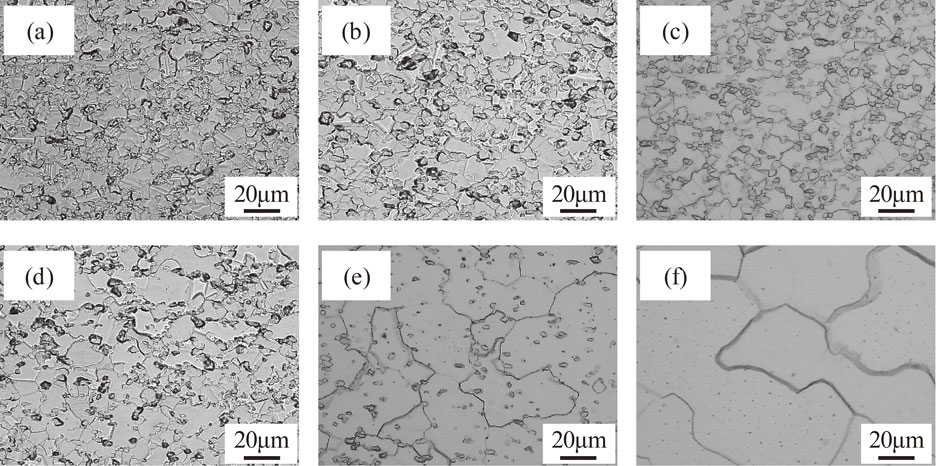Fig. 6

Optical micrographs of compressed TMW-4M3 under the compression strain of 1.4, strain rate of 0.005 s−1, and compression temperature of (a) 1223 K, (b) 1373 K, (c) 1383 K, (d) 1398 K, (e) 1413 K, and (f) 1433 K.Fig. 7

Optical micrographs of compressed TMW-4M3 under the compression strain of 1.4, compression temperature of 1383 K, and strain rate of (a) 0.0005 s−1, (b) 0.005 s−1, and (c) 0.05 s−1.Fig. 8

Compression temperature dependency of dynamic recrystallization grain size.Fig. 9

Strain rate dependency of dynamic recrystallization grain size.

Here, it is known that the Zener-Hollomon parameter, characteristic value of isothermal forging process, and DDRX have the following relationship,

 \begin{equation} D_{\text{DRX}} = A_{\text{DRX}}Z^{- n_{\text{DRX}}}, \end{equation} (5)
where ADRX and nDRX are material constants. This equation shows that forging in a large Z condition, at low temperature, and at a fast strain rate results in small DDRX. In the process design of isothermal forging, the main parameters are the strain rate and forging temperature. By using eq. (5), we can predict the dynamically recrystallized grain size just after the isothermal forging process. Therefore, we have developed numerical modelling of the dynamically recrystallized grain size on the basis of eq. (5). Here, we used the activation energy of steady stress for the calculation of the Zener-Hollomon parameter because DDRX in the general isothermal forging process is defined toward the fully dynamically recrystallized microstructure. The relationship between DDRX and Z of TMW-4M3 is shown in Fig. 10. Generally, a single-phase alloy system has a certain relationship of eq. (5), but TMW-4M3 showed a unique DDRXZ relationship at each compression temperature. This means that even if we compress TMW-4M3 under one certain Z condition, the average value of the dynamically crystallized grain size differs with compression temperature. As stated in Fig. 9, we also found that this ln DDRX − ln Z relationship was grouped in to three regions according to the slope of the approximate lines shown in Fig. 10.

•   Region 1: below 1373 K
•   Region 2: from 1383 K to 1413 K
•   Region 3: 1433 K

Region 1 showed the smallest slope. Moreover, the difference in DDRX under the same Z compression was sufficiently small in this region, so the approximate line seems to converge into one. Region 2 has a larger slope than region 1. In addition, the degree of coarsening was becoming larger with higher temperature. Region 3 indicated the largest slope and a very large grain size. Here, we presumed that the amount of γ′ phase greatly influences DDRX, because it has a pinning effect on matrix grain growth. Then, the measured fraction and average radius of γ′ precipitates for each compression temperature were summarized in Fig. 11. The average sizes of γ′ particles were similar to each other. On the other hand, the γ′ phase fraction was decreasing with higher temperature. The average fraction was more than 25% in region 1, from 15% to 22% in region 2, and less than 1% in region 3. As shown in Fig. 6 and Fig. 7, a lot of γ′ particles were dispersed in the TMW-4M3 microstructure. Therefore, a large amount of γ′ fraction means a large number density, which results in a strong pinning effect on the growth of dynamically recrystallized γ matrix grains.Fig. 10

Relationship between Z and DDRX for all compression conditions. Error bar denotes the standard error.Fig. 11

Measured fraction and average radius of γ′ precipitates after compression test. Error bar denotes the range of values.

We analyzed the grain growth behavior affected by γ′ particles using the following Smith-Zener relationship,21)

 \begin{equation} \skew3\bar{R} = a\frac{\bar{r}}{f^{b}}, \end{equation} (6)
where $\skew3\bar{R}$ is the average grain radius, f is the fraction of pinning particles, and $\bar{r}$ is the average radius of pinning particles. Assuming fixed pinning particles, for example, the material constants a and b were identified as 4/3 and 1, respectively.22) Moreover, many modified models by taking into account such as fraction range, the effects of curvature and interfacial energy of particles have been suggested.2330) They reported different values of a and b in eq. (6). Figure 12 shows the relationship between $\skew3\bar{R}/\bar{r}$ and f in this study. If the recrystallized microstructures of TMW-4M3 obey the general formula, eq. (6), the plots will show the straight line in Fig. 12. However, in particular, in the range of small γ′ fraction, the grain size shifted to the coarse microstructure. Therefore, the recrystallized grain size of TMW-4M3 was affected by both the strain rate and the pinning particles.Fig. 12

Relationship between $\skew3\bar{R}/\bar{r}$ and f.

3.3 DDRX prediction model under pinning effect

We thought that the pinning effect in region 1 was large enough in any temperature conditions, and the difference in grain size for each temperature was quite small. The volume fraction in this region was almost same. However, the contribution of Z was obvously confirmed in Fig. 10. To include the effect of strain rate conditions, one certain DDRXZ relationship was applied to this region, which enables us to predict the relationship between forging conditions and resulting grain sizes. Using the method of least squares, we derived the following prediction formulae,

 \begin{equation} \text{Region 1}\quad D_{\text{DRX}} = 51.4 \times Z^{- 0.0577}. \end{equation} (7)
In region 3, the γ′ phase was quite small and the microstructures were almost γ single phase. Therefore, the pinning effect is negligible, and the conventional DDRXZ relationship seems to be available. From the experimental results shown in Fig. 10, we identified the material constants of eq. (5) as follows,
 \begin{equation} \text{Region 3}\quad D_{\text{DRX}} = 72403 \times Z^{- 0.227}. \end{equation} (8)
As for region 2, the pinning effect was becoming smaller than that of region 1 with decreasing volume fraction of γ′ precipitates. Here, the difference of this pinning effect also affected the microstructures, not only the Z value. Here, by taking the natural log of eq. (5), we obtained the following relationship,
 \begin{equation} \ln D_{\text{DRX}} = \ln A_{\text{DRX}} - n_{\text{DRX}}\ln Z. \end{equation} (9)
The γ′ fraction dependency of the intercept term ln ADRX and the slope nDRX were investigated for the compression temperature of 1383 K, 1398 K, and 1413 K in region 2. From Fig. 10, each ln ADRX was derived as 4.91, 5.25 and 6.35, respectively. Moreover, each nDRX was obatained as 0.084, 0.090 and 0.096, respectively. These results are summarized in Fig. 13. The intercept terms ln ADRX and the slope nDRX were fitted well by quadratic and linear functions, respectively. Then, we identified the material constants using the following expression,
 \begin{equation} \ln A_{\text{DRX}} = 590.4 \times f^{2} - 247.3 \times f + 30.8, \end{equation} (10)

 \begin{equation} n_{\text{DRX}} = - 0.219 \times f + 0.131. \end{equation} (11)
The comparison of DDRX between the experimental results and prediction model calculated by eqs. (7)(11) are shown in Fig. 14. The predicted DDRX agrees quite well with the experimental results in the whole range of isothermal compression conditions by applying not only the conventional Z dependent prediction model but also the new formulae considering the pinning effect through the γ′ fraction. The error in this model is within 12% for DDRX, which is sufficiently accurate for the actual forging process design.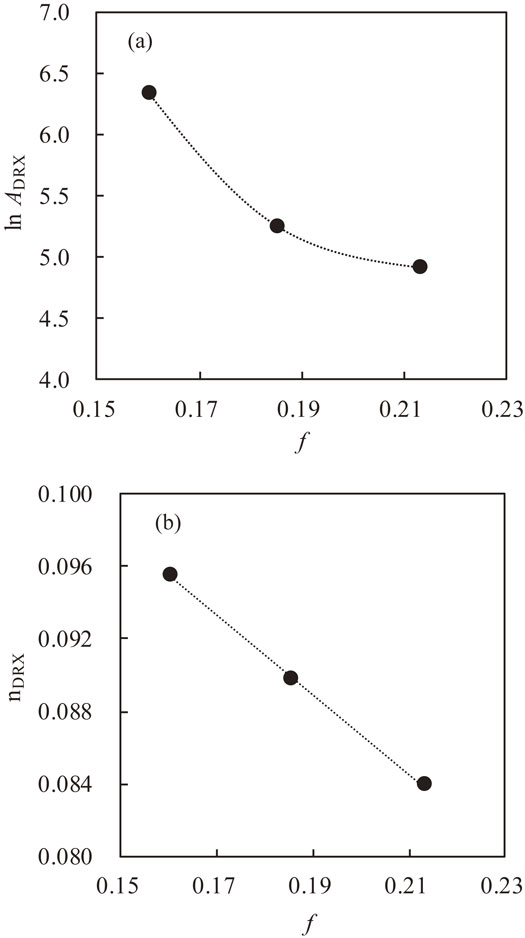Fig. 13

The γ′ fraction, f, dependency of material constants of (a) ln ADRX and (b) nDRX.Fig. 14

Prediction accuracy of developed numerical model for dynamic recrystallization grain size considering pinning effect.

Finally, we summarize our DDRX prediction model. In the dynamically crystallized microstructure system where the pinning effect is significant, the relationship between DDRX and the Zener-Hollomon parameter of the isothermal forging condition is shown as

 \begin{equation} D_{\text{DRX}} = A_{\text{DRX}}(f)Z^{- n_{\text{DRX}}(f)}. \end{equation} (12)
Unlike in the case of conventional modelling, the material constants ADRX and nDRX are dependent on the γ′ fraction and are patterned into the following three groups by the influence rate of the pinning effect.

1. I.    Pinning effect is large enough and dominant for grain boundary migration
 \begin{equation} A_{\text{DRX}} = A_{1},\quad n_{\text{DRX}} = n_{1}. \end{equation} (13)
2. II.    Both pinning effect and Z parameter are considered
 \begin{equation} \ln A_{\text{DRX}} = A_{2}f^{2} + A_{2}'f + A_{2}'',\quad n_{\text{DRX}} = n_{2}f + n_{2}'. \end{equation} (14)
3. III.    Pinning effect is quite small or negligible
 \begin{equation} A_{\text{DRX}} = A_{3},\quad n_{\text{DRX}} = n_{3}. \end{equation} (15)

4. Conclusion

In this paper, the high temperature deformation behavior and the microstructure evolution of Ni–Co base superalloy TMW-4M3 during the isothermal forging process were experimentally and numerically analyzed. Based on the results, the following findings can be summarized:

1. (1)    The flow stress curve of TMW-4M3 shows dynamic recrystallization characteristics with peak stress σp and steady stress σs by compressing under a temperature ranging from 1223 K to 1433 K and strain rate ranging from 0.0005 s−1 to 0.05 s−1. This flow stress can be predicted using the Zener-Hollomon parameter. The specific relationships are as follows,
 \begin{equation*} Z = \dot{\varepsilon}\exp (613600/RT) = 1.07 \times 10^{14} \times \sigma_{\text{p}}^{3.92}, \end{equation*}

 \begin{equation*} Z = \dot{\varepsilon}\exp (410300/RT) = 2.19 \times 10^{7} \times \sigma_{\text{s}}^{3.64}. \end{equation*}
2. (2)    The dynamically recrystallized microstructures are influenced by the pinning effect of γ′ precipitates, not only the Z condition. By introducing the γ′ dependency on the material constants of the conventional DDRXZ relationship, we can predict dynamically recrystallized microstructures after isothermal forging, within an error of 12% for DDRX and 0.3 for the grain size number.

REFERENCES

© 2020 The Japan Institute of Metals and Materials
Top